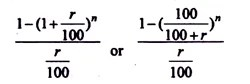# Different Methods of Calculation of Cash Price

In some cases, die cash price is not given. Since the assets purchased cannot be capitalized at more than the cash price, it will be necessary to find out what it is. The way to proceed is to take up die final instalment first and to deduct interest from it. Interest for one year can be found out by multiplying the sum due at the end of the year by the formula Rate of Interest / 100 + Rate of Interest.

Suppose A owes B Rs 100 the interest being 15%. At the end of one year B will have to pay Rs 115 out of which Rs 15 is for interest. Hence, 15/115 of the sum due at the end of the year will be interest. Deducting interest, the sum due in the beginning of the year can be ascertained. This will also be the amount due at the end of the last but one year after paying the annual instalment. The total of these two will give the total sum due at the end of the last but one year.

That year’s interest can again be ascertained by multiplying the total amount due by the formula:Rate of Interest/100 + Rate of Interest

The cash price can also be calculated, if the annual payments are uniform by the formula:

Where r is the rate of interest per cent per annum and n is the number of years over which payment is to be made. This really amounts to finding out the present value of the amount to be paid or received, taking into account the concerned rate of interest. Tables are available for ready calculation.

Example:

On 1st April, 2008, Bihar Collieries obtained a machine on the hire purchase system, the total amount payable being Rs 2, 50,000. Payment was to be made Rs 50,000 down and the balance in four annual installments of Rs 50,000 each. Interest charged was at the rate of 15 per cent. At what value should the machine be capitalized?

Solution:

If amount due in the beginning of a year is Rs 100, interest for the year will be Rs 15 and the amount of instalment due at the end of the year will be Rs 115. Thus, interest is 15/115 or 3/23 of the amount due at the end of each year.

Keeping this in mind, the cash price of the machine can be calculated in the following manner:Alternatively, the present value at 15% per annum of one rupee received annually at the end of four years is Rs 2-85498. Thus, the present value of Rs 50,000 is Rs 50,000 x 2.85498 = Rs 1, 42,749. To this, we add down payment of Rs 50,000. Therefore, the cash price is Rs 1, 42,749 + Rs 50,000 = Rs 1, 92,749.#### intactone

View all posts by intactone →
error: Content is protected !!